# How To Prepare NSEP For Ray Optics

###### ByRamraj Saini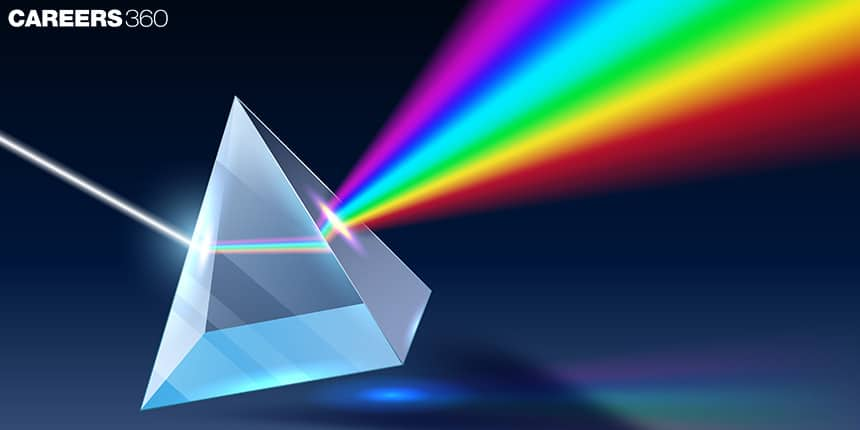###### Synopsis

Optics is an important unit for National Standard Examination in Physics (NSEP). The unit optics can be divided into two parts, Ray Optics, and Wave Optics. The syllabus, important formulas, previous year NSEP questions and study materials to prepare Ray Optics for NSEP are discussed here.###### Synopsis

Optics is an important unit for National Standard Examination in Physics (NSEP). The unit optics can be divided into two parts, Ray Optics, and Wave Optics. The syllabus, important formulas, previous year NSEP questions and study materials to prepare Ray Optics for NSEP are discussed here.

Optics is part of NCERT Class 12, Physics syllabus. The unit Optics is divided into two chapters, Ray Optics And Optical Instruments and Wave Optics. Total weightage of the unit Optics in Past six years is 14 per cent out of which 11 per cent questions are from Ray Optics. Read on to know the syllabus, formulas, some previous year NSEP questions and study materials for Ray Optics

## NSEP Syllabus For Ray Optics

The topics that fall under NSEP syllabus for Ray Optics are: Reflection of light, spherical mirrors, mirror formula, refraction of light, total internal reflection(TIR) and its applications, optical fibres, refraction at spherical surfaces, lenses, thin lens formula, lensmaker's formula, power of a lens, magnification,, combination of thin lenses in contact, prism, refraction through prism, scattering of light and its application, optical instruments: microscopes and astronomical telescopes (reflecting and refracting) and their magnifying powers.

## Ray Optics Important Formulas

The formulas from Ray Optics Chapter are listed here. These may be helpful in solving NSEP questions and NSEP previous year papers.

 Formula Description 1/v+1/u=1/f-Mirror Equation v-object distanceu- image distance from the pole of the mirror.f- focal lengthf=R/2, R-radius of curvature Magnification -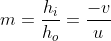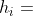height of the image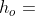height of the object If a prism of refractive index n2is placed in a medium with refractive index n1. then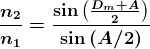A- angle of the prismDm- angle of minimum deviation Thin lens formula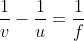Lens makers formula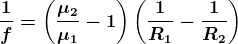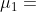the refractive index of the medium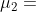the refractive index of the lens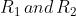are the radius of curvature Power of a lens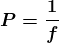P -powerf - focal length in metres For a a simple microscope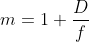For a compound microscope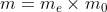D = 25 cm is the least distance of distinct vision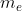is magnification of the eyepiece.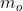is magnification of the objective. Incase of a telescope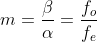? is the angle subtended by eyepiece at the imageɑ is the angle subtended at the eye by the object

## NSEP Previous Year Questions From Ray Optics

Questions from the topic reflection, refraction and optical instruments are covered here. All the topics of Ray Optics are important and questions can be expected from any of the topics. So preparing the entire chapter is a must. There is no possibility of skipping.

Q-1-A small fish, 4 cm below the surface of a lake, is viewed through a thin converging lens of focal length 30 cm held 2 cm above the water surface. Refractive index of water is 1.33. The image of the fish from the lens is at a distance of (NSEP 2017)

(a) 10 cm (b) 8 cm (c) 6 cm (d) 4 cm

Q-2- A horizontal ray of light passes through a prism of refractive index 1.5 and apex angle 4o and then strikes a vertical plane mirror placed to the right of the prism. If after reflection, the ray is to be horizontal, then the mirror must be rotated through an angle (NSEP 2017)

(a) 1 o clockwise (b) 1 o anticlockwise (c) 2 o clockwise (d) 2 o anticlockwise

Q-3- Rays from an object immersed in water (µ = 1.33) traverse a spherical air bubble of radius R. If the object is located far away from the bubble, its image as seen by the observer located on the other side of the bubble will be(NSEP 2017)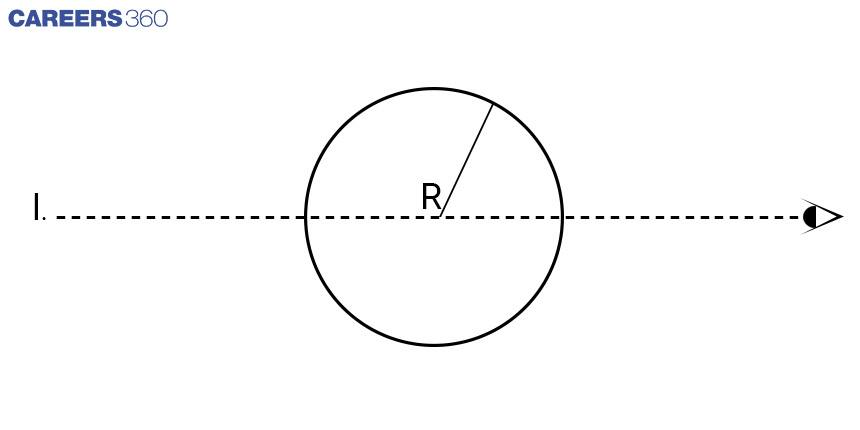(a) virtual, erect and diminished (b) real, inverted and magnified (c) virtual, erect and magnified (d) real, inverted and diminished

Q-4- The aperture diameter of a plano-convex lens is 6 cm and its thickness is 3 mm. If the speed of light through its material is v = 2 108 m/s, the focal length of the lens is(NSEP 2018)

(a) 40 cm. (b) 35 cm. (c) 30 cm. (d) 20 cm.

Q-5-A thin hollow equiconvex lens, silvered at the back, converges a beam of light parallel to the principal axis at a distance 0.2 m. When filled with water (n=4/3 ), the same beam will be converged at a distance of (NSEP 2018)

(a) 0.40 m. (b) 0.20 m. (c) 0.12 m. (d) none of the above

Q-6- Q-2- A converging lens of focal length 40 cm is fixed at 40 cm in front of a screen. An object placed 120 cm from the fixed lens is required to be focused on the screen by introducing another identical lens in between. The second lens should be placed at a distance x from the object where x is(NSEP 2018: One or more option correct)

(a) 40 cm. (b) 50 cm. (c) 140 cm. (d) 150 cm.

Q-7- The optical powers of the objective and the eyepiece of a compound microscope are 100 D and 20 D respectively. The microscope magnification is equal to 50 when the final image is formed at d = 25 cm i.e., the least distance of distinct vision. If the separation between the objective and the eyepiece is increased by 2 cm, the magnification of the microscope will be(NSEP 2021)

(a) 62 (b) 50 (c) 38 (d) 25

Also, Read| How To Prepare Magnetic Effects Of Current

## NSEP Study Materials For Ray Optics

• NCERT Class 12 Physics chapter Ray Optics And Optical Instruments

• NCERT Exemplar problems from Ray Optics And Optical Instruments

• NSEP previous year papers

• Electromagnetism
• Optics
• Natural philosophy
• Atomic, molecular, and optical physics
• Physical phenomena
• Electrodynamics
• Equipment
• Geometrical optics

## Careers360 helping shape your Career for a better tomorrow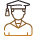#### 250M+

Students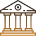#### 30,000+

Colleges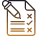#### 500+

Exams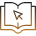E-Books